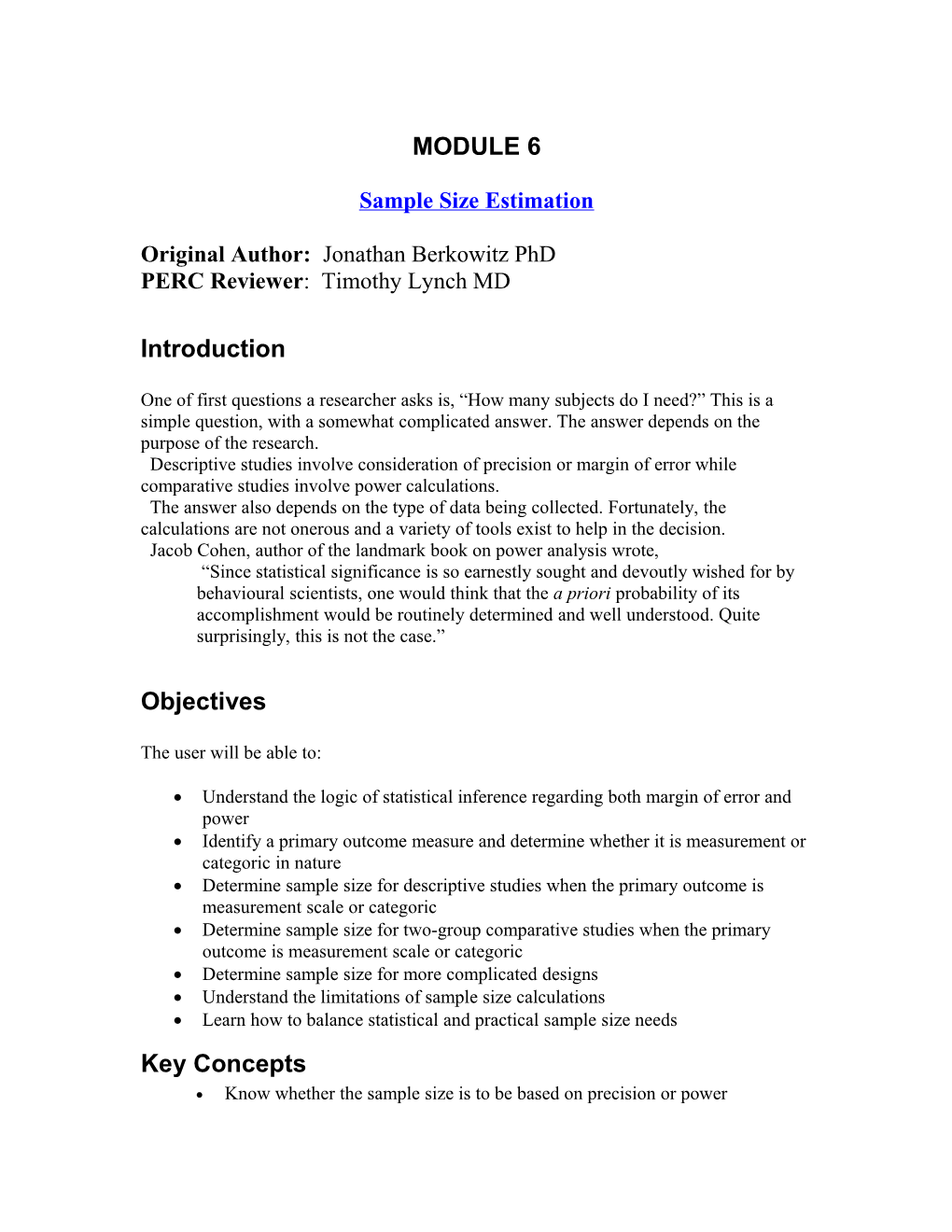# Sample Size Estimation## MODULE 6

Sample Size Estimation

Original Author: Jonathan Berkowitz PhD

PERC Reviewer: Timothy Lynch MD

## Introduction

One of first questions a researcher asks is, “How many subjects do I need?” This is a simple question, with a somewhat complicated answer. The answer depends on the purpose of the research.

Descriptive studies involve consideration of precision or margin of error while comparative studies involve power calculations.

The answer also depends on the type of data being collected. Fortunately, the calculations are not onerous and a variety of tools exist to help in the decision.

Jacob Cohen, author of the landmark book on power analysis wrote,

“Since statistical significance is so earnestly sought and devoutly wished for by behavioural scientists, one would think that the a priori probability of its accomplishment would be routinely determined and well understood. Quite surprisingly, this is not the case.”

## Objectives

The user will be able to:

• Understand the logic of statistical inference regarding both margin of error and power
• Identify a primary outcome measure and determine whether it is measurement or categoric in nature
• Determine sample size for descriptive studies when the primary outcome is measurement scale or categoric
• Determine sample size for two-group comparative studies when the primary outcome is measurement scale or categoric
• Determine sample size for more complicated designs
• Understand the limitations of sample size calculations
• Learn how to balance statistical and practical sample size needs

## Key Concepts

• Know whether the sample size is to be based on precision or power
• Learn how many respondents are needed for survey design
• Learn how to compute sample size based on power
• Strategies for minimizing sample size
• Find suitable “sample size calculators” on the web

## Activities

• Decide on a primary outcome measure which will be the basis for sample size determination
• Determine sample sizes necessary for various research scenarios
• Be able to apply these calculations to your research project

Sample Size Calculators on the Web

In increasing order of complexity:

Rollin Brant’s simple but effective sample size calculators

Deals with epidemiological applications.

Nicely designed java-applets

An excellent index page for many other on-line statistical tools.

Overview of Sample Size Determination